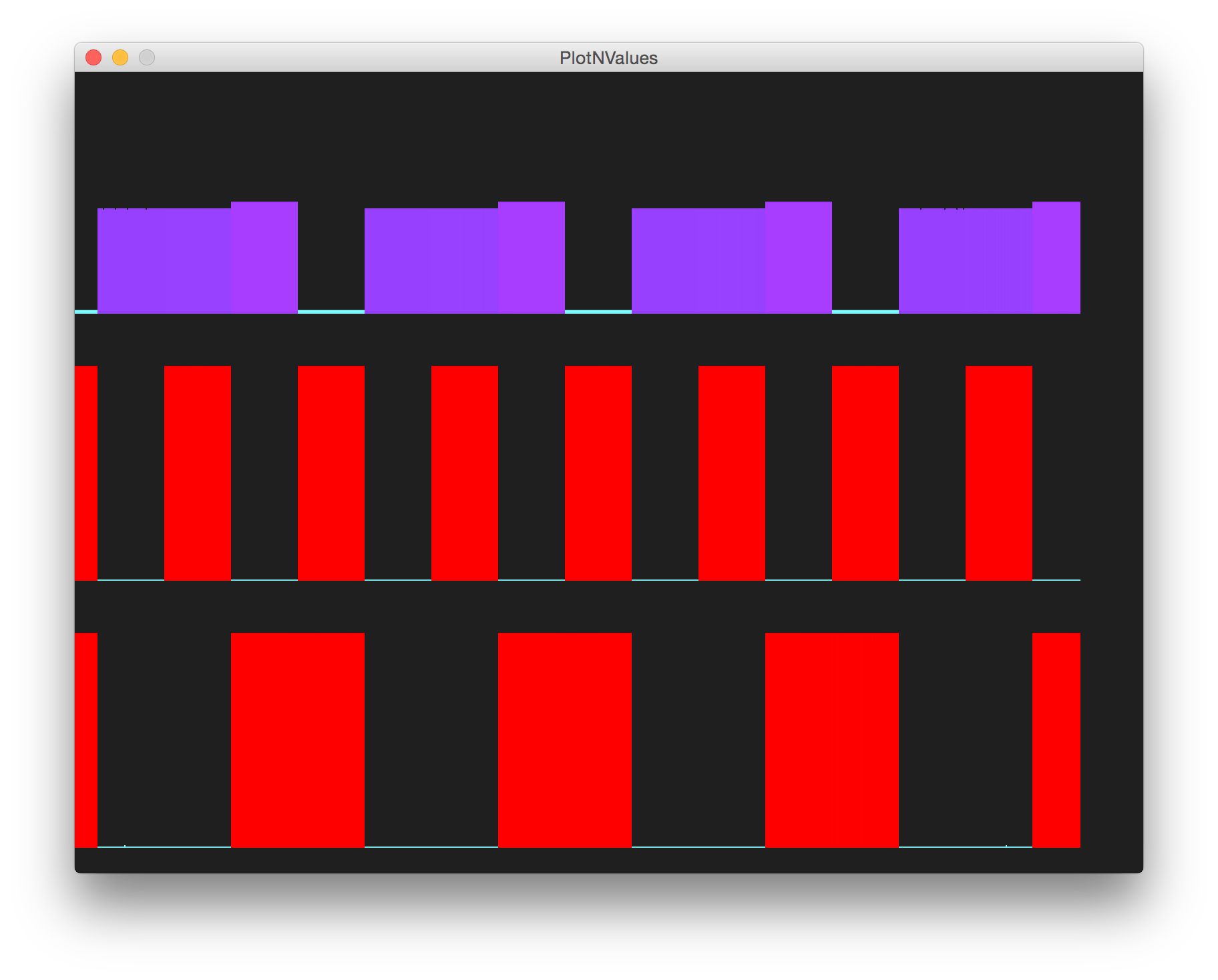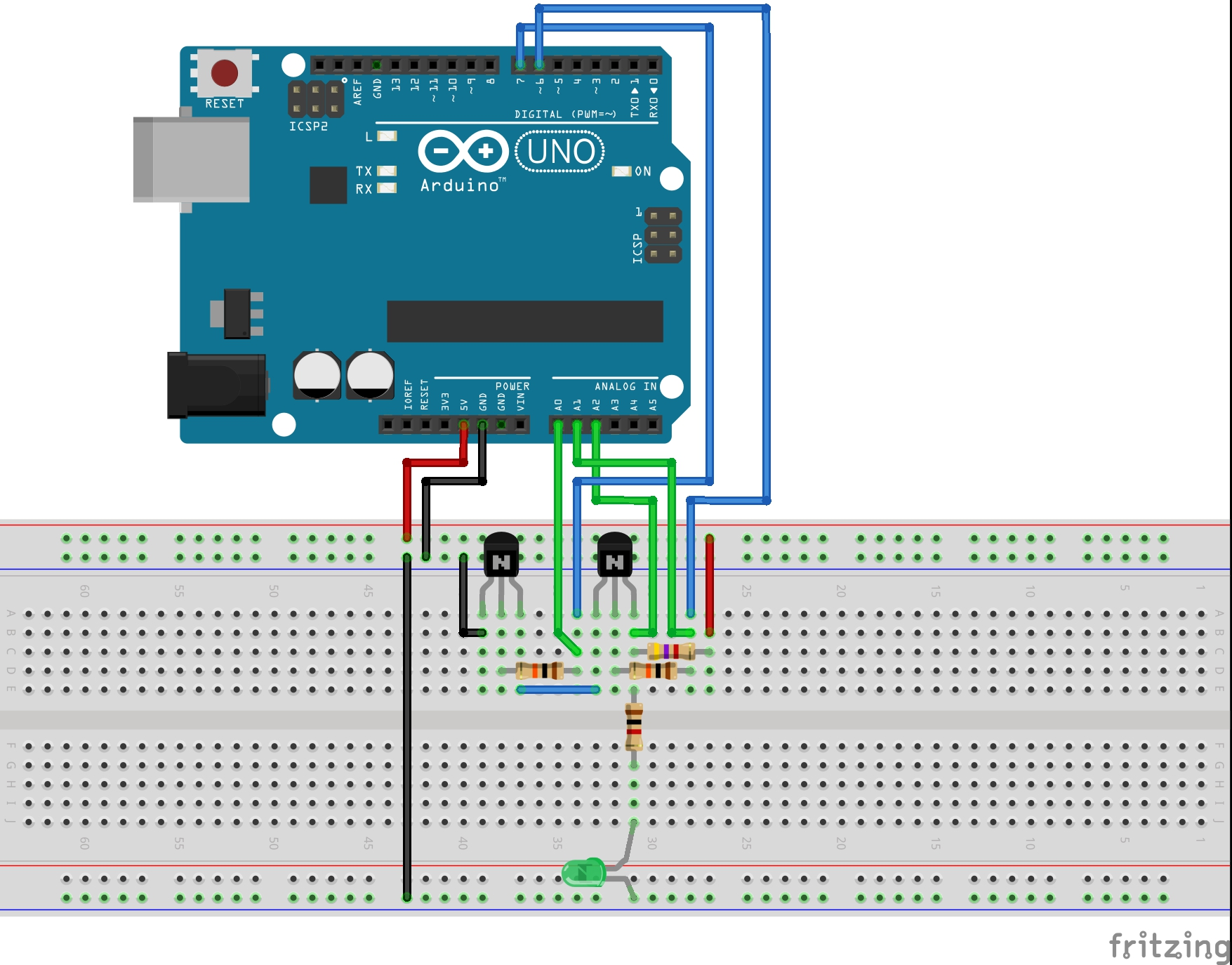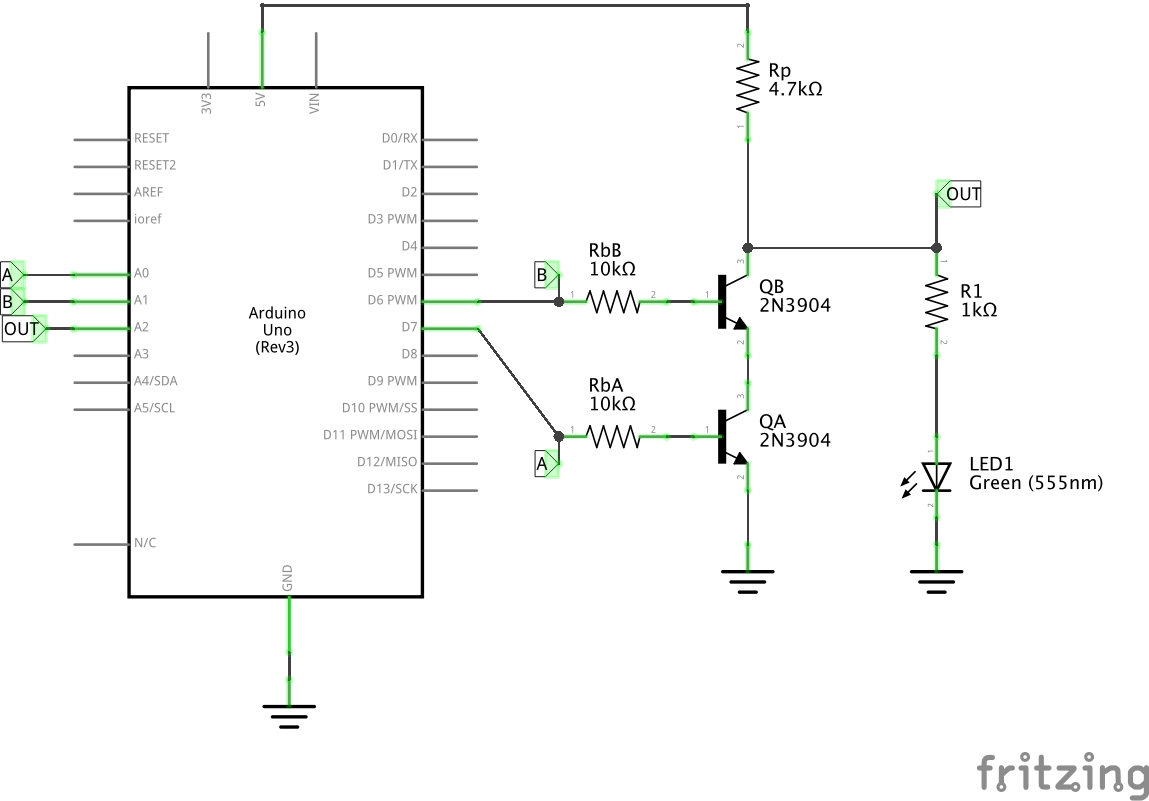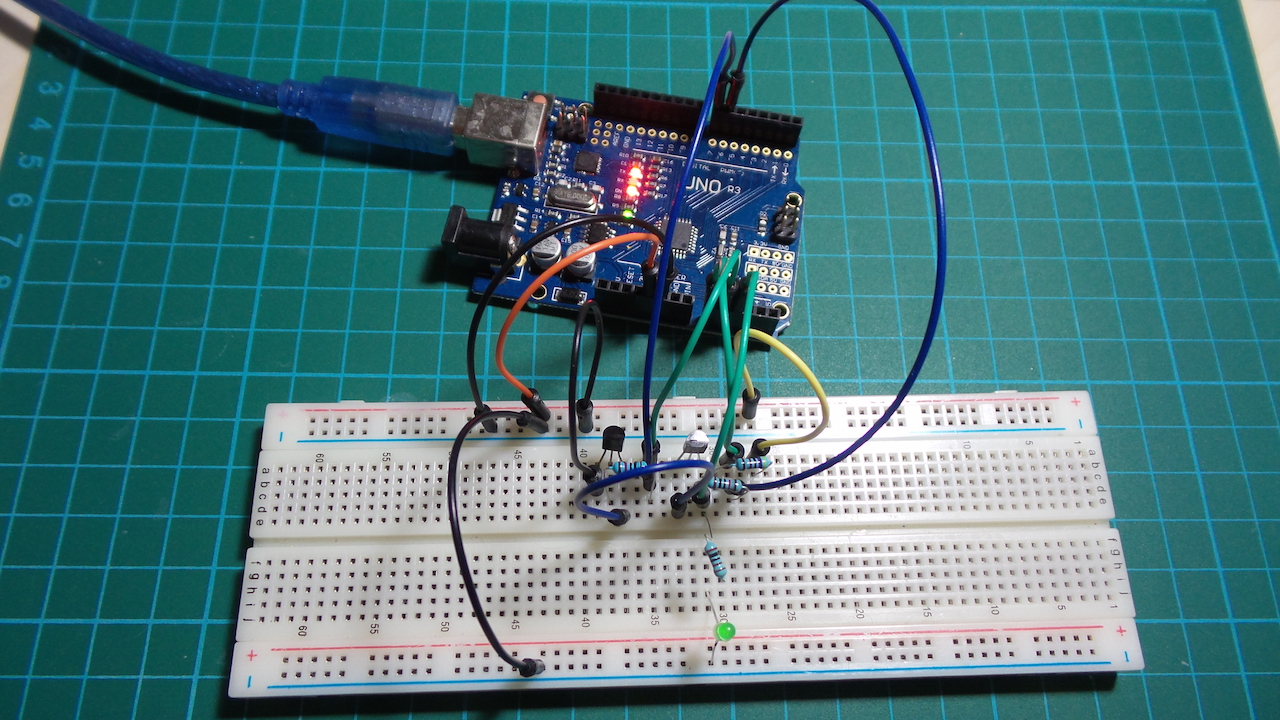# #023 ResistorTransistorLogic/NAND

Test the basic resistor-transistor logic NAND gate.

Resistor–transistor logic (RTL) is a class of digital circuits built using resistors as the input network and bipolar junction transistors (BJTs) as switching devices. RTL is the earliest class of transistorized digital logic circuit and is largely obsolete now.

It is interesting to see how various logic gate may be realised with BJTs and resistors alone. Here is the basic NAND gate.

This is the “classic” two-transistor NAND gate, often used when describing the fundamentals of digital logic. Given two ideal transistors (NPN) with collector-emitter in series, we treat them as switches: only when both are on should there be a low output.

A B OUT
0 0 1
0 1 1
1 0 1
1 1 0

However, that’s the idealised view. In practice things are a little messier. In particular:

• A pull-down resistor (Rp) is required to get a clean “off”, else the input would be floating.
• With the lower transistor on, we can see partial activation. This is because we are applying a voltage to the base while the collector is not driven, so the base is appearing on the emitter. We have to work around this problem be definining the threshold for “high” to be above this voltage.

Here’s a sample trace. The lower two traces are the signal inputs, and the upper trace is the output of the gate.The Arduino is not really a core part of the circuit. It only does the following:

• provides +5V power supply (for convenience)
• automates the toggling of the driving inputs
• measures the input and output voltages for plotting

## Construction

The circuit uses a digital output pins to sequence inputs to the gate. Three analog input pins are used to read the two input signals and the resuting output, with the values is echoed to the Arduino serial port for plotting with PlotNValues (a simple Processing sketch).